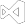# OCR a Region of an ImageOCR a Region of an Image
``````using IronOcr;

var Ocr = new IronTesseract();

using (var Input = new OcrInput())
{
// a 41% improvement on speed by specifiying a pixel region
var ContentArea = new System.Drawing.Rectangle() { X = 215, Y = 1250, Height = 280, Width = 1335 };

Console.WriteLine(Result.Text);
}``````
``````Imports IronOcr

Private Ocr = New IronTesseract()

Using Input = New OcrInput()
' a 41% improvement on speed by specifiying a pixel region
Dim ContentArea = New System.Drawing.Rectangle() With {
.X = 215,
.Y = 1250,
.Height = 280,
.Width = 1335
}